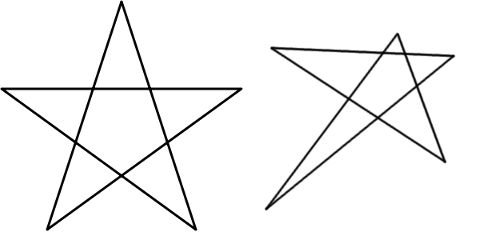# Sum of Angles in Star Polygons

Futility Closet recently posted a nice puzzle about the sum of the angles in the “points” of a star polygon.It’s easy to show that the five acute angles in the points of a regular star, like the one at left, total 180°.

Can you show that the sum of these angles in an irregular star, like the one at right, is also 180°?  (link)

A clever proof is shown, but what I would consider the standard proof is clever, simple, and beautifully generalizable.

Consider the star pentagon below.By the exterior angle theorem, we have$\angle 1 = \alpha_1 + \beta$$\angle 2 = \alpha_1 + \theta$

So$\angle 1 + \angle 2 = \alpha_1 + \alpha_1 + \beta + \theta = \alpha_1 + 180 \textdegree$

where the last equality follows from the triangle angle sum formula.

Do this for each of the five “points” and sum the equations$2( \angle 1 + \angle 2 + \angle 3 + \angle 4 + \angle 5) = \sum \alpha_i + 5 \times 180 \textdegree$

Now, the sum of the interior angles of any pentagon, regular or not, is$540 \textdegree$, so this becomes$2 \times 540 \textdegree = \sum \alpha_i + 5 \times 180 \textdegree$

And so, a little arithmetic gives us$\sum \alpha_i = 180 \textdegree$

For stars of this type, where the points are formed by intersecting two sides of an n-gon that are separated by exactly one side, this method generalizes beautifully.  The above equation becomes$2 \times (n-2) \times 180 \textdegree = \sum \alpha_i + n \times 180 \textdegree$

which simplifies to$\sum \alpha_i = (n-4) \times 180 \textdegree$

In particular, we see that when = 5, we have that$\sum \alpha_i = 180 \textdegree$.  And one of the best things about having a formula like this is asking questions like “What happens when n = 4?” and “What happens when = 3?”!

Now, there are other types of star polygons.  For example, if you start with an octagon, extend the sides, and consider the intersections of two sides that themselves are separated by exactly two sides of the octagon, you get something that looks like this.While the formula above doesn’t apply to this star, a similar technique does.  The big difference is that, instead of the star’s points being attached an an n-gon (a pentagon, in the first example), this star’s points are attached to another star polygon!  There are lots of fun directions to go with this exploration.

Categories: GeometryTeaching#### Anonymous · May 7, 2015 at 1:50 pm

I came up with an alternate solution.

Join all of the star’s vertices to draw the enclosing pentagon of the star. We have that the exterior pentagon has 5 angles, which sum up to 540 degrees, and it includes the 5 angles we want plus additional stuff. We note that the additional stuff is 2 of the 3 angles of 5 triangles. We can add the remaining angles that we need to get the 5 triangles. These 5 angles are the same 5 angles of the interior pentagon, so we are adding 540 degrees. So we get

Sum of 5 angles at the points + stuff + 5 angles from the interior pentagon = 540 + 540
Sum of 5 angles at the points + 5 * 180 = 1080
Sum of 5 angles at the points = 180

or symbolically, using the same notation from your diagram,

\sum a_i + stuff = 540
\sum a_i + stuff + (measure of angles 1, 2, 3, 4, 5) = 540 + 540
\sum a_i + 5*180 = 1080
\sum a_i = 180

I hope this explanation was clear enough. I also came up with this solution before looking at the clever solution on the page.#### Anonymous 2 · December 25, 2015 at 7:45 am

well, there is another solution with me…..
see, we know that the total sum of exterior angles of any polygon = 360degrees (2*180)
now the sum of the exterior angles of the inner pentagon is also 360 degree and we can take it in 2 directions(one is in the direction of theta and the and the other in the direction of beta)
so the total sum of exterior angles is 360*2 (180*4)
but these exterior angles are angles of triangles which contain all the five angles which we need,
therefore,
sum of all five angles + the other 2 angles of all triangles = 180*5 (there are 5 triangles)
But the sum of other 2 angles of all triangles = total sum of exterior angles in 2 ways
Therefore,
sum of all 5 angles + 360*2 =180*5
sum of all 5 angles = 180*5 – 360*2
= 180 degrees
Hence, PROVED…..

Follow